## one object has an excess of 74 electrons and another object has 50 less electrons than protons. If the two objects are separated by a distan

Question

one object has an excess of 74 electrons and another object has 50 less electrons than protons. If the two objects are separated by a distance of .35m, what is the electric force between the two? Is it attractive or repulsive?

in progress 0
3 months 2021-08-03T17:32:12+00:00 1 Answers 3 views 0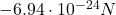, attractive

Explanation:

The charge of one electron is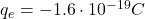And the first object has an excess of 74 electrons,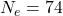, so the net charge of the first object is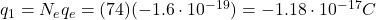The charge of one proton is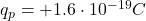And the 2nd object has 50 less electrons than protons,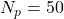, so the net charge of the 2nd object is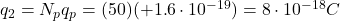The electric force between two charged objects is given by Coulomb’s law: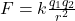where

k is the Coulomb’s constant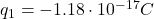is the charge on the 1st object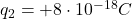is the charge on the 2nd object

r = 0.35 m is the distance between the two objects

Substituting, we find: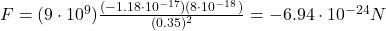And the negative sign indicates that the force is attractive (in fact, the electric force between two charges of opposite sign is attractive, while the force between two charges of same sign is repulsive)